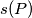### MEMO 2007 ekipno problem 6

Kvaliteta:
Avg: 2,5
Težina:
Avg: 6,0
For a set$P$ of five points in the plane, no three of them being collinear, let$s(P)$ be the numbers of acute triangles formed by vertices in$P$.
Find the maximum value of$s(P)$ over all such sets$P$.
Izvor: Srednjoeuropska matematička olimpijada 2007, ekipno natjecanje, problem 6